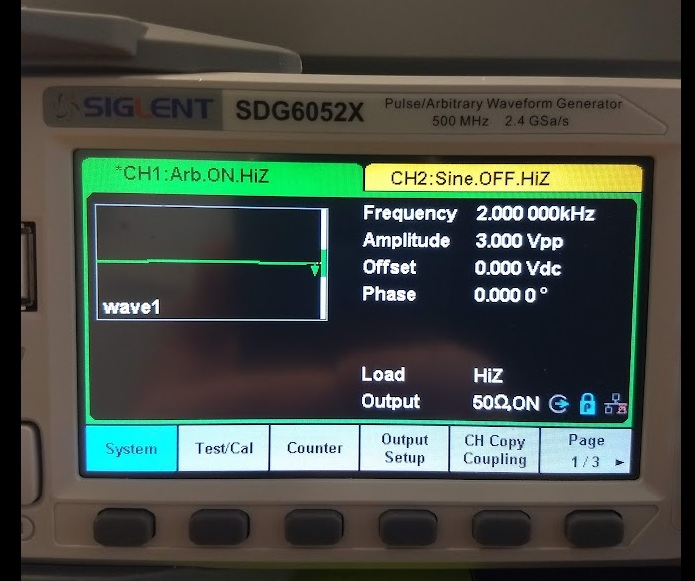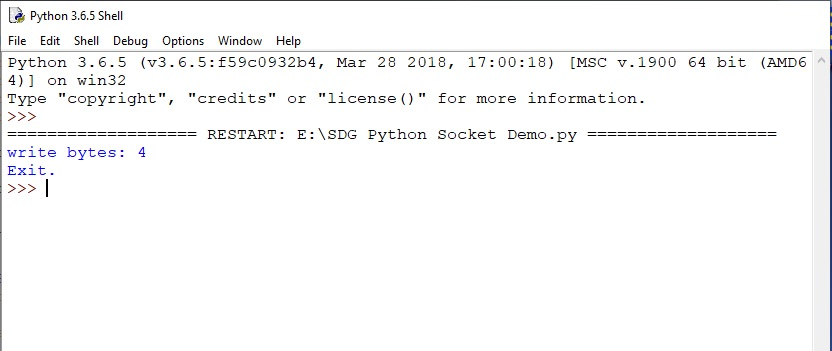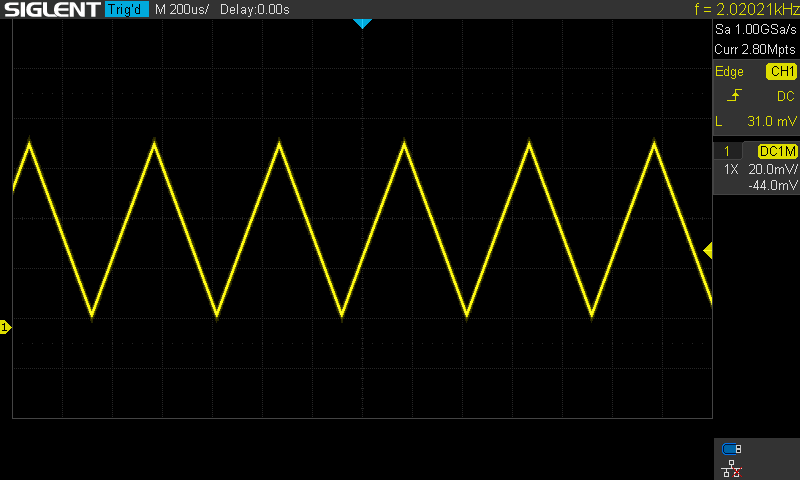# Programming Example: SDG waveform creation with Python and Sockets (no VISA)

December 13, 2022

Here is a programming example using Python and Sockets over LAN to create a two-point waveform.

Sockets via LAN can be helpful if you wish or are unable to use the VISA library.

Here is a picture of the data once it has been loaded into the SDG:Here is a picture of the generator output on the controlling computer:Here is the generator output on an oscilloscope:SDG Python Socket Demo

```import socket
import sys
import time
import binascii

remote_ip = "192.168.1.84"
port = 5025
count = 0

wave_points = [0x8000, 0x3f06]

for i in range (1000):
wave_points = wave_points + [0x8000, 0x3f06]

def SocketConnect():
try:
s = socket.socket(socket.AF_INET, socket.SOCK_STREAM)
except socket.error:
print('Fail to creat socket.')
sys.exet();
try:
s.connect((remote_ip, port))
except socket.error:
print('failed to connect to ip' + remote_ip)
return s

def SocketQuery(Sock, cmd):
try:
Sock.sendall(cmd)
time.sleep(1)
except socket.error:
print('Send failed')
sys.exit()

def SocketSend(Sock, cmd):
try:
cmd = cmd + '\n'
Sock.sendall(cmd.encode('latin1'))
time.sleep(1)
except socket.error:
print('Send failed.')
sys.exit()

def SocketClose(Sock):
Sock.close()
time.sleep(.300)

def create_wave_file():
f = open('wave1.bin','wb')
for a in wave_points:
b = hex(a)
b = b[2:]
len_b = len(b)
if(0 == len_b):
b = '0000'
elif(1 == len_b):
b = '000' + b
elif(2 == len_b):
b = '00' + b
elif(3 == len_b):
b = '0' + b
c = binascii.a2b_hex(b)
f.write(c)
f.close()

def main():
global remote_ip
global port
global count

create_wave_file()
s = SocketConnect()

f = open('wave1.bin', 'rb')
data1 = data.encode('latin1')
with open('wave2.bin', 'wb') as f1:
f1.write(data1)

print('write bytes:', len(data))

data = str(data)

SocketSend(s,"C1:WVDT WVNM,wave1,FREQ,2000.0,AMPL,3.0,OFST,0.0,PHASE,0.0,WAVEDATA,%s"%(data))
SocketSend(s,'C1:ARWV NAME,wave1')
f.close()
SocketClose(s)
print('Exit.')

if __name__ == '__main__':
proc = main()

```